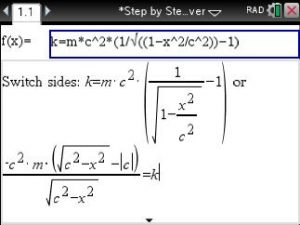# Step by Step Equation Solver – How to enter and solve some tricky equations

Say we need to solve :Since the Step by Step Equation Solver solves for the variable x we just replace v by x to enter as shown below:Note: for the square root symbol press:  CTRL   +   x^2

Now view steps until the final answer is derived.

Posted on Categories solver, tips & tricks